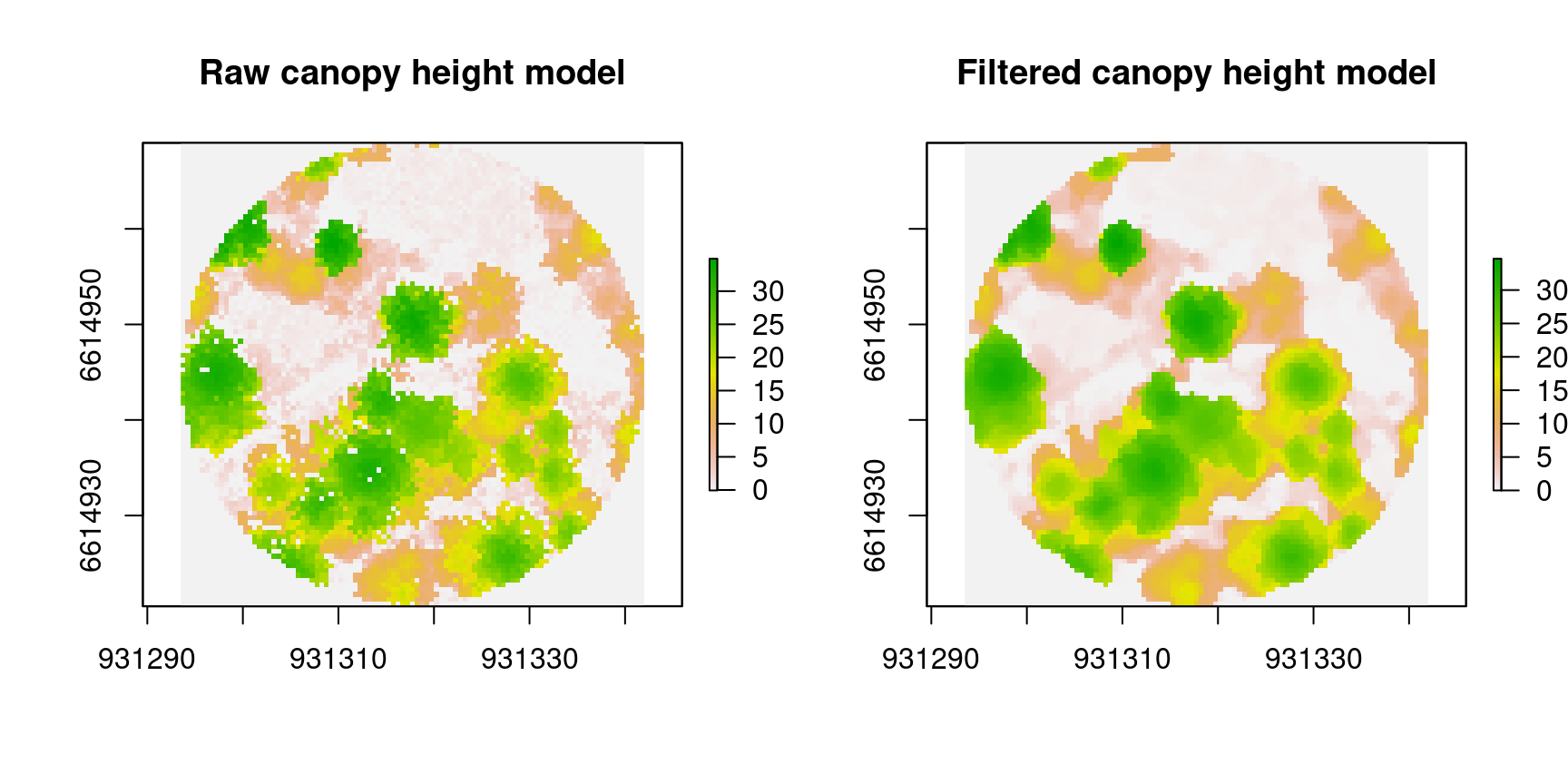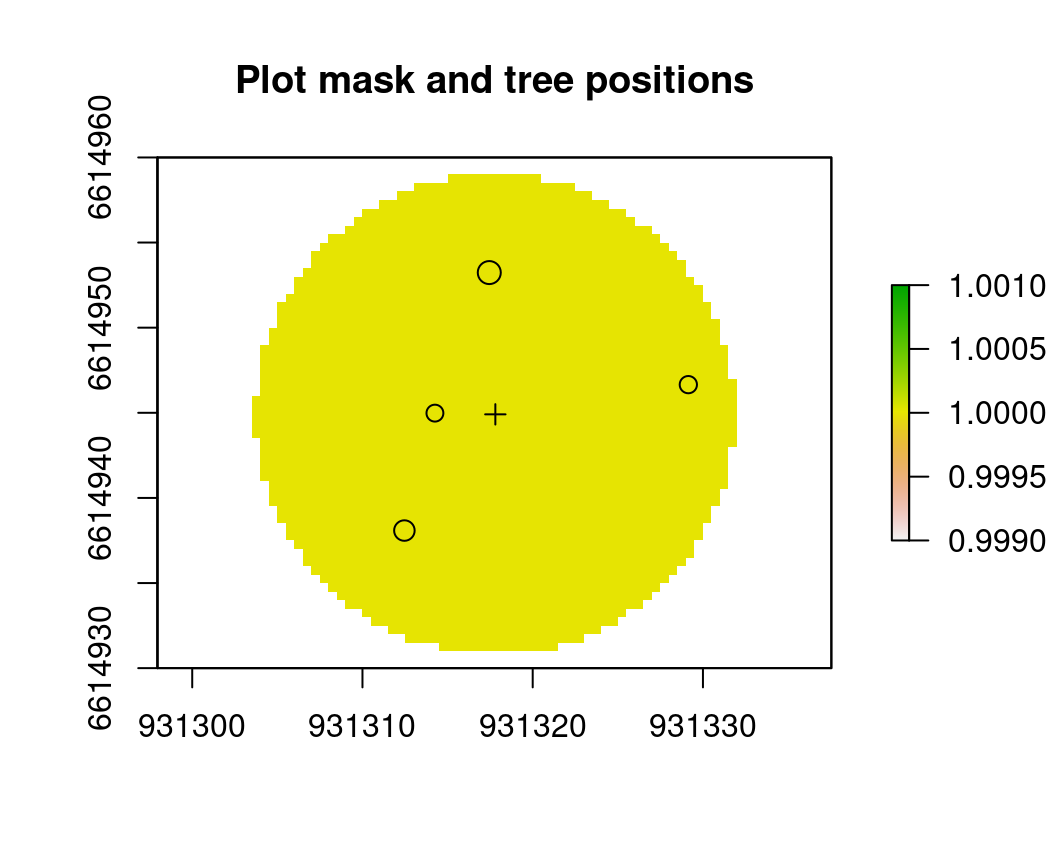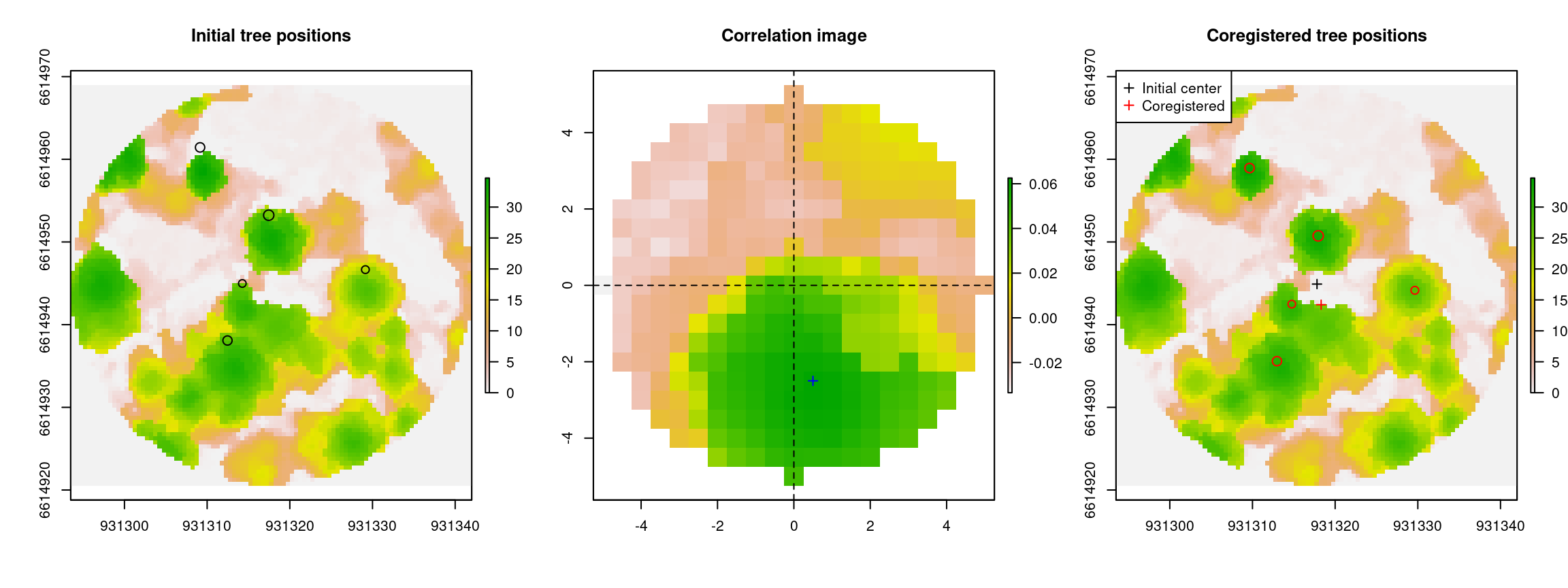This workflow compares a canopy height model (CHM) derived from airborne laser scanning (ALS) data with tree positions inventoried in the field, and then proposes a translation in plot position for better matching. The method is described in Monnet and Mermin (2014). Here it is exemplified with circular plots, but it can be applied to any shape of field plots. The workflow is based on functions from packages `lidaRtRee` and `lidR`. Example data were acquired in the forest of Lac des Rouges Truites (Jura, France).

Licence: CC BY / Source page

## Material

### Field data

The study area is a part of the forest of Lac des Rouges Truites. 44 plots have been inventoried, 15 are available for testing. Plots have a 14.10 m radius. A data.frame `p` contains the positions of the center of plots. Attributes are:

• `placette`: plot id
• `Xtheo` and `Ytheo`: XY coordinates initially sampled when preparing the field inventory
• `XGPS` and `YGPS`: XY coordinates recorded in the field with a GNSS receiver during the field inventory
• `Prec`: GNSS precision in meter specified by the receiver
• `dist`: horizontal distance between the sample and recorded coordinates.
``````# load plot coordinates (data.frame with lines corresponding to the las objects)
``````##   placette    Xtheo   Ytheo     XGPS    YGPS     ZGPS Prec     dist
## 1        6 930422.7 6615047 930423.1 6615046 1087.803  2.1 1.502383
## 2       12 930521.4 6615046 930517.3 6615047 1082.799  1.6 4.161719
## 3       22 930720.9 6615249 930722.1 6615245 1089.593  0.3 3.980429``````

On each plot, five trees which were considered suitable for coregistration (vertical trunk, high and peak-shaped crown, well separated from neighboring trees) have been positioned relatively to the plot center. From the XY coordinates recorded by the GNSS receiver and the relative coordinates (slope, distance, azimuth), the XY coordinates of those trees are computed. Data.frame `ap` contains the following attributes:

• `plac`: plot id
• `n`: tree id
• `dia`: tree diameter in cm
• `distR`: slope distance from the plot center to tree center, in m
• `azimutG`: azimuth from the plot center to the tree center, in grades
• `pente.`: slope from plot center to tree center, in grades
• `XYZGPS`: coordinates of the plot center
• `xyz`: coordinates of the tree center
• `d`: horizontal distance between plot center and tree in m
``````# load inventoried trees (data.frame with plot id info )
``````##   plac   n  ess dia distR azimutG pente. Rem     XGPS    YGPS     ZGPS        x
## 1    6 223 ABAL  54  9.02       9    -29     930423.1 6615046 1087.803 930424.2
## 2    6 224 ABAL  48  9.18     304     52     930423.1 6615046 1087.803 930415.0
## 3    6 225 ABAL  45  7.91     214     52     930423.1 6615046 1087.803 930421.6
##         y        z        d
## 1 6615054 1085.291 8.663071
## 2 6615046 1092.038 8.144650
## 3 6615039 1091.452 7.017885``````

### ALS data

Airborne laser scanning data on the study area is part of a campaign acquired in 2016 with an airborne RIEGL LMS Q680i sensor. Acquisition was funded by the Région Franche-Comté.

ALS data over the plots is provided as a list of LAS objects in `rda` file.

``````# load point cloud over reference plots (list of las objects)

Display point cloud of plot 1.

``````# plot point cloud
plot(las[])``````

The code to extract LAS objects from a directory containing the LAS files is (code corresponding to parameters in the next paragraph has to be run beforehand):

``````# create catalog of LAS files
cata <- lidR::catalog("/directory_with_classified_laz_files/")
# set coordinate system
sp::proj4string(cata) <- sp::CRS(SRS_string = "EPSG:2154")
# extract LAS data on plot extent
las <- lidR::clip_circle(cata, p\$XGPS, p\$YGPS, p.radius + b.size + 5)
# normalize heights if point cloud are not already normalized
las <- lapply(las, function(x) {lidR::normalize_height(x, lidR::tin())})
# save as rda file for later use
save(las, file="./data/coregistration/lasCoregistration.rda")``````

## Parameters

Several parameters have to be specified for optimal results.

``````# vegetation height threshold for removal of high values
hmax <- 50
# field plot radius for computation of pseudo-CHM on the inventory area
# raster resolution for image processing
r.res <- 0.5
# maximum distance around initial position to search for coregistration
b.size <- 5
# increment step to search for coregistration (should be a multiple of resolution)
s.step <- 0.5``````

## Coregistration of one plot

### Canopy height model

The first step is to compute the canopy height model from the ALS data, and remove artefacts by thresholding extreme values and applying a median filter.

``````# Choose a plot as example
i <- 10
# compute canopy height model
chm <- lidaRtRee::points2DSM(las[[i]],res=r.res)
# apply threshold to canopy height model (CHM)
chm[chm>hmax] <- hmax
# fill NA values with 0
chm[is.na(chm)] <- 0
# apply median filter (3x3 window) to CHM
chmfilt <- lidaRtRee::demFiltering(chm,"Median",3, sigmap=0)\$non.linear.image
# plot canopy height model
par(mfrow=c(1,2))
raster::plot(chm, main="Raw canopy height model")
raster::plot(chmfilt, main="Filtered canopy height model")``````### Plot mask from tree inventory

The trees corresponding to the plot are extracted, and a plot mask is computed from the plot center and radius.

``````# plot centre
centre <- p[i,c("XGPS","YGPS")]
# extract plot trees
trees <- ap[ap\$plac==p\$placette[i],]
# create raster with plot extent
r <- lidaRtRee::circle2Raster(centre\$XGPS, centre\$YGPS, p.radius, resolution=r.res)
# keep only trees with diameter information
trees <- trees[!is.na(trees[,"dia"]),]
# create plot mask
c(centre\$XGPS, centre\$YGPS)),
# replace 0 by NA
# specify projection
# display plot mask
# add tree positions
points(trees[,c("x","y")], cex=trees[,"dia"]/40)
# add plot center
points(centre, pch=3)``````### Compute correlation and identify optimal translation

First the function computes the correlation for different possible translations of the plot center inside the buffer specified by the user, and outputs an image of correlation values between the ALS CHM and the pseudo CHM. Second this image is analyzed to identify which translation yields the highest correlation. The function outputs a list which first element is the correlation image, and the second one the corresponding statistics.

``````# compute correlation image
coreg <- lidaRtRee::coregistration(chmfilt, trees[,c("x", "y", "dia")], mask=r.mask,
buffer=b.size, step=s.step, dm=2, plot=F)
# correlation image statistics
round(coreg\$local.max, 2)``````
``````##     max1 dx1  dy1 dx2 dy2 ratiomax1max2 rmedloc1 rmedloc2 rquanta rquantb
## 75% 0.06 0.5 -2.5 2.5   4          3.97     1.07      1.2    1.49     8.6``````

The maximum of in the correlation image is located at (0.5, -2.5), given by attributes `dx1` and `dy1`.

``````par(mfrow=c(1,3))
raster::plot(chmfilt, main="Initial tree positions")
# display initial tree positions
graphics::points(trees\$x, trees\$y, cex=trees\$dia/40)
# display correlation image
raster::plot(coreg\$correlation.raster, main="Correlation image")
# plot local maximum
graphics::points(coreg\$local.max\$dx1, coreg\$local.max\$dy1, pch=3, col="blue")
abline(h=0, lty=2); abline(v=0, lty=2)
#
raster::plot(chmfilt, main="Coregistered tree positions")
# display coregistered tree positions
graphics::points(trees\$x+coreg\$local.max\$dx1, trees\$y+coreg\$local.max\$dy1,
cex=trees\$dia/40, col="red")
# display initial plot center
graphics::points(p[i, c("XGPS","YGPS")], pch=3)
# display coregistered plot center
graphics::points(p\$XGPS[i]+coreg\$local.max\$dx1, p\$YGPS[i]+coreg\$local.max\$dy1, pch=3,
col="red")
graphics::legend("topleft", c("Initial center", "Coregistered"), pch=3,
col=c("black", "red"))````````````# export as pdf
# dev.copy2pdf(file=paste("Coregistration_",p\$placette[i],".pdf",sep=""))``````

## Batch processing

The following code processes several plots using multi-core computing. It is based on packages `foreach` and `doParallel` for parallel computing.

``````# number of cores to use for parallel processing
doParallel::registerDoParallel(cores=4)
library(foreach)``````

### CHMs computation

First CHMs are calculated for each point cloud contained in the list.

``````l.chm <- foreach::foreach (i=1:length(las), .errorhandling="remove") %dopar%
{
# compute CHM
chm <- lidaRtRee::points2DSM(las[[i]],res=r.res)
# apply threshold to canopy height model (CHM)
chm[chm>hmax] <- hmax
# fill NA values with 0
chm[is.na(chm)] <- 0
# apply median filter (3x3 window) to CHM
chmfilt <- lidaRtRee::demFiltering(chm,"Median",3, sigmap=0)[]
return(chmfilt)
}``````

### Trees lists extraction and plot masks computation

``````l.field <- foreach::foreach (i=1:length(las), .errorhandling="remove") %dopar%
{
# plot centre
centre <- p[i,c("XGPS","YGPS")]
# extract plot trees
trees <- ap[ap\$plac==p\$placette[i],]
# create raster with plot extent
r <- lidaRtRee::circle2Raster(centre\$XGPS, centre\$YGPS, p.radius, resolution=r.res)
# keep only trees with diameter information
trees <- trees[!is.na(trees[,"dia"]),]
# create plot mask
c(centre\$XGPS, centre\$YGPS)),
# replace 0 by NA
# specify projection
#
}``````

### Correlation images and corresponding statistics computation

Then the correlation image and corresponding statistics are computed for each plot.

``````l.coreg <- foreach::foreach (i=1:length(las), .errorhandling="remove") %dopar%
{
buffer=b.size, step=s.step, dm=2, plot=F)
}``````

Finally results for all plots are combined in a single data.frame.

``````translations <- foreach::foreach (i=1:length(las), .combine=rbind,
.errorhandling="remove") %dopar%
{
# create data.frame with coregistration results and new plot coordinates
data.frame(plotid=p\$placette[i], X.cor=p\$XGPS[i]+l.coreg[[i]]\$local.max\$dx1,
Y.cor=p\$YGPS[i]+l.coreg[[i]]\$local.max\$dy1, l.coreg[[i]]\$local.max)
}
#
``````##      plotid    X.cor   Y.cor max1  dx1 dy1 dx2  dy2 ratiomax1max2 rmedloc1
## 75%       6 930422.1 6615047 0.06 -1.0   1   2 -4.5          4.79     1.07
## 75%1     12 930516.8 6615045 0.07 -0.5  -2  -4  3.0          2.58     1.13
## 75%2     22 930721.1 6615247 0.06 -1.0   2   0 -5.0          4.64     1.09
##      rmedloc2 rquanta rquantb
## 75%      1.31    1.70   12.80
## 75%1     1.51    2.62    4.77
## 75%2     1.36    1.88    8.37``````

### Export graphics as pdf files

``````for (i in 1:length(las))
{
# CHM
pdf(file=paste("Coregistration_",p\$placette[i],".pdf",sep=""))
raster::plot(l.chm[[i]], asp=1)
# display initial tree positions
graphics::points(l.field[[i]]\$trees\$x, l.field[[i]]\$trees\$y,
cex=l.field[[i]]\$trees\$dia/40)
# display coregistered tree positions
graphics::points(l.field[[i]]\$trees\$x+l.coreg[[i]]\$local.max\$dx1,
l.field[[i]]\$trees\$y+l.coreg[[i]]\$local.max\$dy1,
cex=l.field[[i]]\$trees\$dia/40, col="red")
# display initial plot center
graphics::points(p[i, c("XGPS","YGPS")], pch=3)
# display coregistered plot center
graphics::points(p\$XGPS[i]+l.coreg[[i]]\$local.max\$dx1,
p\$YGPS[i]+l.coreg[[i]]\$local.max\$dy1, pch=3, col="red")
graphics::legend("topleft", c("Initial", "Coregistered"), pch=15,
col=c("black", "red"))
# export as pdf
dev.off()
}``````

### Add coregistered plot positions and update tree coordinates

Optimized plot center positions are added to the original data.

``````p <- base::merge(p, translations[,c("plotid", "X.cor", "Y.cor")], by.x="placette",
by.y="plotid")
``````##   placette    Xtheo   Ytheo     XGPS    YGPS    ZGPS Prec dist    X.cor   Y.cor
## 1        6 930422.7 6615047 930423.1 6615046 1087.80  2.1 1.50 930422.1 6615047
## 2       12 930521.4 6615046 930517.3 6615047 1082.80  1.6 4.16 930516.8 6615045
## 3       22 930720.9 6615249 930722.1 6615245 1089.59  0.3 3.98 930721.1 6615247``````

Tree positions are corrected to account for new center position.

``````# add plot center coregistered coordinates to trees data.frame
ap <- base::merge(ap, p[,c("placette","X.cor","Y.cor")], by.x="plac", by.y="placette")
# compute new tree coordinates from coregistered plot center
dummy <- lidaRtRee::polar2Projected(ap\$X.cor, ap\$Y.cor, ap\$ZGPS, ap\$azimutG/200*pi,
ap\$distR, atan(ap\$pente./100), 1.55/180*pi,
-2.2/180*pi,0)
ap\$X.cor <- dummy\$x
ap\$Y.cor <- dummy\$y
# save new table
# write.table(round(p.cor,3),file="coregistered_plots.csv", row.names=F,sep=";")``````

### Analysis of GPS errors

Graphics on the difference between initial and corrected positions are displayed. The mean difference is -0.85m in the X axis and -0.9m in the Y axis. `p.values` of a t.test are respectively 0.03 and 0.12. Mean absolute distance is 2.09m with a standard deviation of 0.79m.

``````par(mfrow=c(1,2))
# plot position difference with additionnal jitter to visualize same points
plot(jitter(p\$X.cor- p\$XGPS), jitter(p\$Y.cor-p\$YGPS), asp=1, col="black",
main="Corrected-Initial position", xlab="X difference", ylab="Y difference")
abline(v=0, lty=2); abline(h=0, lty=2)
# Distance between initial and corrected
hist(sqrt((p\$X.cor- p\$XGPS)^2+ (p\$Y.cor-p\$YGPS)^2), main="Histogram of distances",
xlab="Absolute distance corrected-initial", ylab="Number of plots")``````